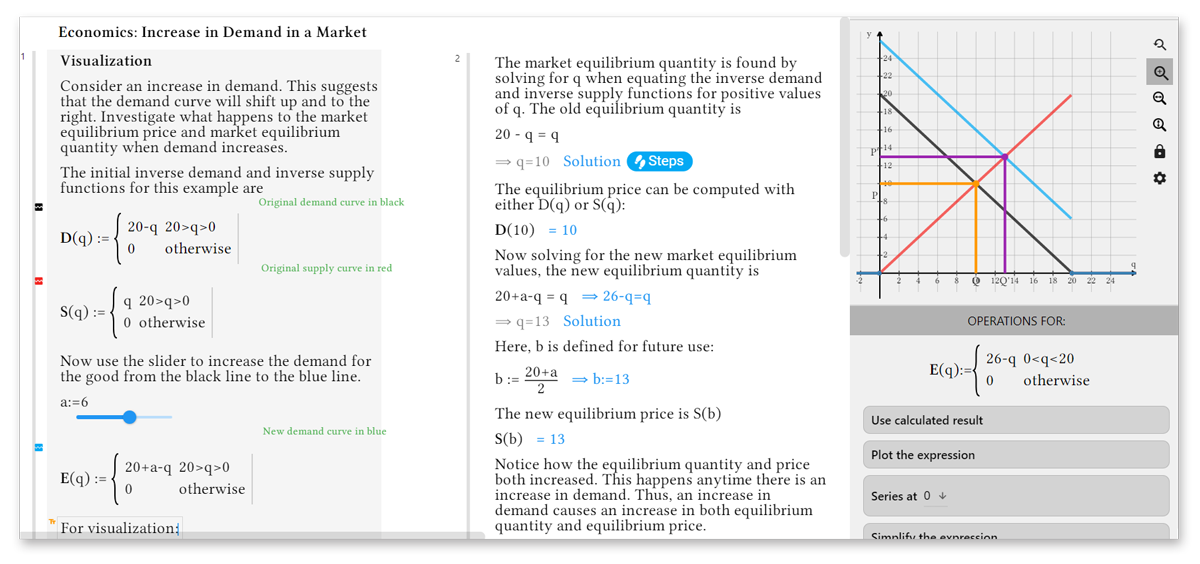Maple Learn for Economics - Maplesoft

# Maple Learn for Economics

Maple Learn is a tool for exploring concepts, doing calculations, and creating rich, online course content.

In economics courses, you use formulas, graphs, and calculations to explore market factors and trends that impact production, distribution and consumption. But all too often, the mechanics of performing the calculations and producing the graphs becomes a barrier to your student’s learning, preventing them from seeing and comprehending the bigger picture. Maple Learn makes it simple for students to perform the mathematics they need, so instead of being a barrier, the calculations and graphs help your students grasp and work with important economics concepts more deeply and easily.

Maple Learn is:

… an open canvas for exploring concepts and doing calculations

The Maple Learn canvas gives you an interactive environment where you can introduce ideas, answer questions, explore what-if scenarios, and walk students through problems. In Maple Learn, you can:

• Calculate formulas
• Graph 2-D and 3-D expressions automatically, and sketch curves using points
• Find limits, solve partial derivatives, manipulate matrices, find means, and much more, all at the click of a button
• Include problem statements, conclusions, and explanations
• Control parameters through sliders, and watch results and graphs change instantly… an environment that brings static content to life

Maple Learn brings learning to life, supporting content that combines:

• The rich presentation of textbook content, combining text, formulas, graphs, and images
• The guided explorations of interactive learning objects that deepen conceptual understanding
• The open-endedness of a notebook where students try things for themselves

In Maple Learn, you can provide your students with lessons, explorations, examples, worked solutions, practise quizzes, homework, and more.

Whether you want to create your own content, or customize existing documents on economics topics, Maple Learn is the easiest way to create rich, interactive content and share it with your economics classes online.

Want to see your own content in Maple Learn?
Let us show you how Maple Learn can bring YOUR lesson to life.